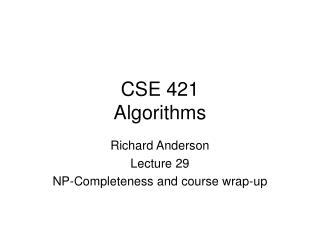DownloadDownload PresentationCSE 421 Algorithms

# CSE 421 Algorithms

Télécharger la présentation## CSE 421 Algorithms

- - - - - - - - - - - - - - - - - - - - - - - - - - - E N D - - - - - - - - - - - - - - - - - - - - - - - - - - -
##### Presentation Transcript

1. CSE 421Algorithms Richard Anderson Lecture 29 NP-Completeness and course wrap-up

2. Today • NP-completeness • Reductions • Problem Thresholds • Number problems • What is NP? • What we don’t know about NP-completeness • Course summary • Evaluations

3. NP-Completeness Reductions • If X is NP-Complete, Y is in NP, and X <P Y, then Y is NP-Complete

4. Hamiltonian Circuit, Hamiltonian Path How do you show that Hamiltonian Path is NP-Complete?

5. Local Modification • Convert G to G’ • Pick a vertex v • Replace v by v’ and v’’ • If (u,v) is an edge, include edges (u, v’), (u, v’’) • G’ has a Hamiltonian Path from v’ to v’’ iff G has a Hamiltonian Circuit

6. HamPath <P DirHamPath How do you show that Directed Hamiltonian Path is NP-Complete?

7. Problem definition • Given a graph G, does G have an independent set? • Given a graph G, does G have an independent set of size 7? • Given a graph G, and an integer K, does G have an independent set of size K?

8. NP-Complete Graph K-coloring Graph 3-coloring Polynomial Graph 2-Coloring Graph Coloring

9. Number Problems • Subset sum problem • Given natural numbers w1,. . ., wn and a target number W, is there a subset that adds up to exactly W? • Subset sum problem is NP-Complete • Subset Sum problem can be solved in O(nW) time

10. Subset sum problem • The reduction to show Subset Sum is NP-complete involves numbers with n digits • In that case, the O(nW) algorithm is an exponential time and space algorithm

11. What is NP? • Problems where ‘yes’ instances can be efficiently verified • Hamiltonian Circuit • 3-Coloring • 3-SAT • Succinct certificate property

12. What about ‘negative instances’ • How do you show that a graph does not have a Hamiltonian Circuit • How do you show that a formula is not satisfiable?

13. What we don’t know • P vs. NP NP-Complete NP = P NP P

14. Stable Matching (2) Models of computation and efficiency (1) Basic graph algorithms BFS, Bipartiteness, SCC, Cycles, Topological Sort (2) Greedy Algorithms (2) Interval Scheduling, HW Scheduling Correctness proofs Dijkstra’s Algorithm (1) Minimum Spanning Trees (2) Recurrences (2) Divide and Conquer Algorithms (3) Closest Pair, FFT Dynamic Programming (7) Weighted interval scheduling, subset sum, knapsack, longest common subsequence, shortest paths Network Flow (5) Ford Fulkerson, Maxflow/mincut, Applications NP-Completeness (3) Course summaryWhat did we cover in the last 29 lectures? Number of lectures on each topic in parentheses